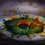# How many programs for the Euler phi?

How many ways can you think of , for writing a program that gives Euler's Totient function ?

Here are 2 that use 2 different definitions of the phi function.

$\mathbf{1.}$ This program uses the basic definition of $\phi(n)$ , which is

### $\phi(n)$ is the number of natural numbers less than $n$, which are coprime to $n$

 1 2 3 4 5 6 7 8 >>> from fractions import gcd >>> def phi(n): """Euler's Totient Function... phi(n)""" li=[] for i in range(n): if gcd(n,i)==1: li.append(i) return len(li) 

Now as we took $\text{gcd}(n,i)=1$ ,

we actually took them coprime, counting all of them and getting them in the list li.

We print len(li) which is length of the list, the number of natural numbers less than $n$, which are coprime to $n$.

$\mathbf{2.}$ This program uses a formula by using primes,

### For a natural number $n$ , we can define $\phi(n)$ as $\phi(n) = n\times \prod _{p\mid n} \Bigl(1-\frac{1}{p}\Bigr)$

or in other words, if $n = p_1^{a_1}p_2^{a_2} ... p_n^{a_n}$, then $\phi(n) = n \Bigl(1-\frac{1}{p_1}\Bigr)\Bigl(1-\frac{1}{p_2}\Bigr)....\Bigl(1-\frac{1}{p_n}\Bigr)$

  1 2 3 4 5 6 7 8 9 10 >>> def checkprime(n): return not(n<2 or any(n%i==0 for i in range(2,int(n**0.5)+1))) >>> def phi(n): """Euler Totient Function... phi(n)""" k=n for i in range(n): if checkprime(i) is True and n%i==0: k= k- k/i return k 

Hence for every prime number $p$ dividing $n$, the program transforms $n\rightarrow n - \frac{n}{p}$ , at the end resulting into the value $\phi(n)$

Anyone knowing any other method is welcome to post it :)Note by Aditya Raut
6 years, 3 months ago

This discussion board is a place to discuss our Daily Challenges and the math and science related to those challenges. Explanations are more than just a solution — they should explain the steps and thinking strategies that you used to obtain the solution. Comments should further the discussion of math and science.

When posting on Brilliant:

• Use the emojis to react to an explanation, whether you're congratulating a job well done , or just really confused .
• Ask specific questions about the challenge or the steps in somebody's explanation. Well-posed questions can add a lot to the discussion, but posting "I don't understand!" doesn't help anyone.
• Try to contribute something new to the discussion, whether it is an extension, generalization or other idea related to the challenge.
• Stay on topic — we're all here to learn more about math and science, not to hear about your favorite get-rich-quick scheme or current world events.

MarkdownAppears as
*italics* or _italics_ italics
**bold** or __bold__ bold
- bulleted- list
• bulleted
• list
1. numbered2. list
1. numbered
2. list
Note: you must add a full line of space before and after lists for them to show up correctly
paragraph 1paragraph 2

paragraph 1

paragraph 2

[example link](https://brilliant.org)example link
> This is a quote
This is a quote
    # I indented these lines
# 4 spaces, and now they show
# up as a code block.

print "hello world"
# I indented these lines
# 4 spaces, and now they show
# up as a code block.

print "hello world"
MathAppears as
Remember to wrap math in $$ ... $$ or $ ... $ to ensure proper formatting.
2 \times 3 $2 \times 3$
2^{34} $2^{34}$
a_{i-1} $a_{i-1}$
\frac{2}{3} $\frac{2}{3}$
\sqrt{2} $\sqrt{2}$
\sum_{i=1}^3 $\sum_{i=1}^3$
\sin \theta $\sin \theta$
\boxed{123} $\boxed{123}$

## Comments

Sort by:

Top Newest

Wow! Still no comments ? Pls wait till the 4th , I'll post a Java solution then :)

- 6 years, 2 months ago

Log in to reply

Hi aditya raut I am preparing for inmo this year what tips can you give ma

- 5 years, 9 months ago

Log in to reply

×

Problem Loading...

Note Loading...

Set Loading...